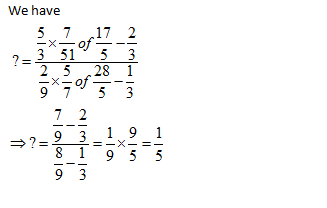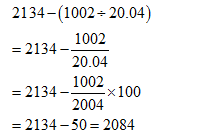• This is an assessment test.
• These tests focus on the basics of Maths and are meant to indicate your preparation level for the subject.
• Kindly take the tests in this series with a pre-defined schedule.

## Basic Maths: Test 12

Congratulations - you have completed Basic Maths: Test 12. You scored %%SCORE%% out of %%TOTAL%%. You correct answer percentage: %%PERCENTAGE%% . Your performance has been rated as %%RATING%%
 Question 1
$\displaystyle 10-\left[ 4-\left\{ 3-\left( 3-3-6 \right) \right\} \right]$ is equal to:
 A 15 B 16 C 14 D 10
Question 1 Explanation:
$\displaystyle \begin{array}{l}p=10-\left[ 4-\left\{ 3-\left( 3-3-6 \right) \right\} \right]\\=10-\left[ 4-\left\{ 3-\left( -6 \right) \right\} \right]\\=10-\left[ 4-\left\{ 3+6 \right\} \right]\\=10-\left[ 4-9 \right]\\=10+5=15\end{array}$
 Question 2
Evaluate:$\displaystyle \frac{-{{\left( 4-6 \right)}^{2}}-3\left( -2 \right)+6}{17-9\div 3\times 5}$
 A $\displaystyle \frac{3}{8}$ B $\displaystyle 7$ C $\displaystyle 4$ D $\displaystyle \frac{7}{4}$
Question 2 Explanation:
$\displaystyle \begin{array}{l}?=\frac{-{{\left( -2 \right)}^{2}}+6+6}{17-15}\\=\frac{-4+12}{2}=\frac{8}{2}=4\end{array}$
 Question 3
Simplify: $\displaystyle \frac{\frac{5}{3}\times \frac{7}{51}of\,\frac{17}{5}-\frac{2}{3}}{\frac{2}{9}\times \frac{5}{7}of\,\frac{28}{5}-\frac{1}{3}}$
 A $\displaystyle \frac{1}{2}$ B $\displaystyle 4$ C $\displaystyle \frac{1}{5}$ D $\displaystyle \frac{1}{4}$
Question 3 Explanation:Question 4
$\displaystyle 3-\left[ 5-\left\{ 2+\left( -5+6-2 \right)2 \right\} \right]$ is equal to
 A -4 B 2 C 0 D -2
Question 4 Explanation:
$\displaystyle \begin{array}{l}p=3-\left[ 5-\left\{ 2+\left( -1 \right)2 \right\} \right]\\=3\times \left[ 5-\left\{ 2-2 \right\} \right]\\=3-\left[ 5-0 \right]\\=3-5=-2\end{array}$
 Question 5
On simplification 2134−(1002/20.04) is equal to:
 A 3029 B 2084 C 2993 D 2543
Question 5 Explanation:Once you are finished, click the button below. Any items you have not completed will be marked incorrect.
There are 5 questions to complete.
 ← List →